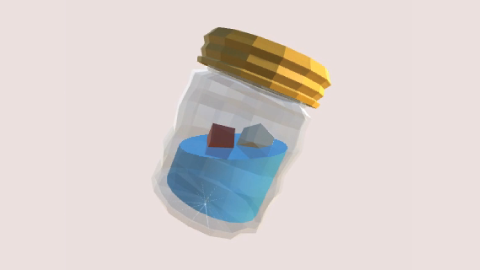##### Shane Celis November 24, 2015 Nov 24, 2015 #math #physics

I’m interested in simulating buoyancy for a game idea I’m exploring. I didn’t expect implementing buoyancy to be difficult since the physics is easy to describe and I’m limiting myself to cuboids, rectangles in 3D. But it led to a math problem that took me some time to crack. Rather than solve and forget, I thought I’d document it as a guide for other game developers who may want to do something similar. There will be a lot of math, but fear not: there’s a kitty at the end!

## The Physics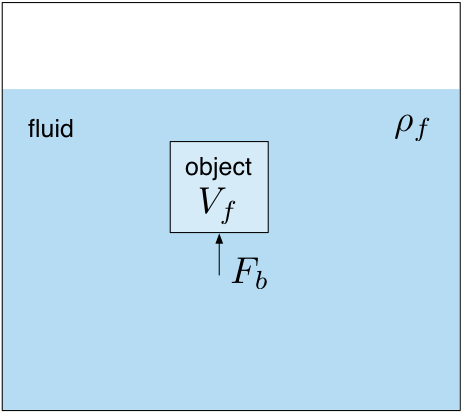Our situation is this, we have an object submerged in a fluid which exerts an upward force, the buoyant force $F_b$. The physics is easy describe mathematically.

$$F_b \propto V_f$$

The buoyant force $F_b$ is proportional to the volume of the displaced fluid $V_f$. The more fluid the object displaces, the greater the buoyant force. We can be more specific.

$$F_b = \rho_f V_f g$$

The buoyant force $F_b$ is equal to the density of the fluid $\rho_f$ times the volume of the displaced fluid $V_f$ and the acceleration of gravity $g = 9.8\, m/s^2$. Another way of saying that is the buoyant force is equal to the weight of the displaced fluid.

So if we have a cube with sides of length $s$ totally submerged in a fluid, the volume of fluid displaced is the same as the volume of the cube $s^3$, and the buoyant force exerted on the cube would be $F_b = \rho_f s^3 g$.

## The Complication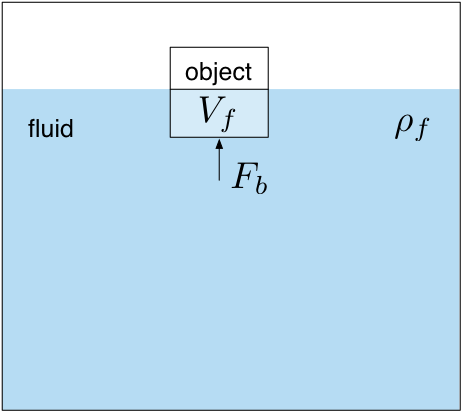The complication we face is the case where the object is not entirely submerged in the fluid. In this situation the volume of the fluid displaced no longer equals the volume of the object.

$$V_f = ?$$

We have to find a way to calculate these partial volumes.

### Easy in 2D, Not in 3D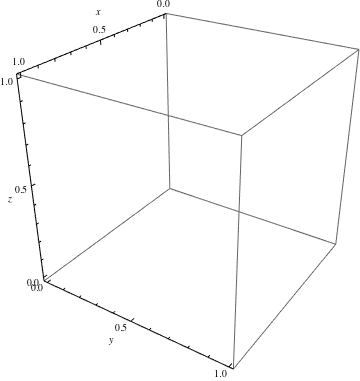In two dimensions, this is relatively easy to solve. The water line cuts the object, and using geometry we can figure out what proportion of the square is under water.

In three dimensions, it’s harder to solve. A plane cuts the object, and geometry is no longer sufficient. We’ve got to use calculus. Yay! (Why is no one else excited?)

#### Voxel Approximation

I should note that we could sidestep calculus and do a voxel approximation, which has the advantage of being simple and easy to code. But this code could be running every physics time step, so performance may be an issue. Still a voxel approximation is a great trick to have in your back pocket. For this case, you’d split up your cube into $m^3$ cubelettes and sum only the cubelettes’ volume that are under the plane. This is also a good way to double check your more sophisticated derivations because it’s simple, easy to code, but tune-able for accuracy: the greater $m$, the more accurate it is. But it’s an $O(m^3)$ algorithm.

## Making It Easier

We can make this problem easier in a couple ways.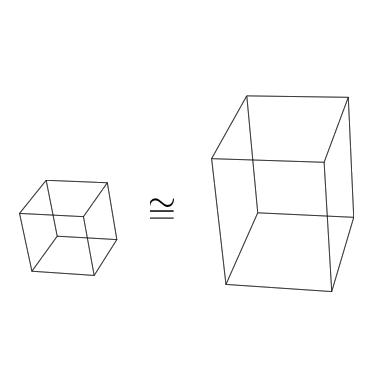• We assume the water line is a plane.
• Assume the components of the normal of the plane are all positive.
• Without loss of generality (I hope), we can solve this problem for a unit cube and with a little transformation we can apply the solution to a cuboid of any dimensions.

## Motivation

With that, I hope I have provided the motivation for why we’re interested in finding the volume of a unit cube below a plane: Because video games. Although cuboids aren’t the most exciting shape, they’re often a good approximation for many shapes. We’ve done the physics. We’ve made our approximations and assumptions. We only have the math left to do.

# Problem: Volume of Unit Cube Below Plane

Determine the volume of a unit cube below a plane with normal $n = (a,b,c)$ and a point $p_0 = (x_0, y_0, z_0)$ where $d = n \cdot p_0$. The unit cube is situated with one corner at the origin $(0,0,0)$ and the other at $(1,1,1)$.

The volume of the unit cube is 1. But let’s setup the problem using calculus for the volume.

$$\int_{y_0}^{y_1} \int_{x_0}^{x_1} \int_{z_0}^{z_1} dz\, dx\, dy$$

Set the limits of integration for our unit cube.

$$\int_0^1 \int_0^1 \int_0^1 dz\, dx\, dy$$

And it equals 1. Now we’re going to change the integration limits $z_1, x_1,$ and $y_1$ to be bounded by the plane.

## The Plane

The equation for a plane is $a x + b y + c z - d = 0$. We need to account for cases where either $a,b,c = 0$. We’ll use a little trick to turn that from eight cases into three. We’re assuming that $a,b,c \ge 0$.

### Case 1: $a,b,c \ne 0$

Let’s find the limit for $z_1$ by solving for $z$ in the plane equation.

$$z = (d - a x - b y)/c$$

So our first integral of the three is

$$\int_0^{(d - a x - b y)/c} dz \text{ .}$$

To find $x_1$ we solve $(d - a x - b y)/c = 0$ for $x$, which is $(d - b y)/a$. (I can’t justify why we’re saying $z=0$ and solving for $x$. I need to refer to my calculus book on triple integrals and volume applications.) We have our second integral.

$$\int_0^{(d - b y)/a} \int_0^{(d - a x - b y)/c} dz\,dx$$

To find $y_1$ we solve $(d - b y)/a = 0$ for $y$, which is $d/b$. This gives us our complete integral expression:

$$V_1 = \int_0^{d/b} \int_0^{(d - b y)/a} \int_0^{(d - a x - b y)/c} dz\,dx\,dy$$ $$V_1 = \frac{d^3}{6 a b c}$$

### Case 2: $b = 0$

Our plane equation is

$$a x + c z - d = 0$$

and we go through the same steps as in case 1.

$$V_2 = \int_0^{1} \int_0^{d/a} \int_0^{(d - a x)/c} dz\,dx\,dy$$ $$V_2 = \frac{d^2}{2 a c}$$

### Case 3: $b = a = 0$

Our plane equation is

$$c z - d = 0$$

and we go through the same steps as in case 1. $$V_3 = \int_0^1 \int_0^1 \int_0^{d/c} dz\, dx\, dy$$

$$V_3 = \frac{d}{c}$$

## Complication

We’ve done our integration. We’re feeling proud of ourselves but there’s a snag. The integration limits aren’t quite as easy as we’ve made ourselves believe. What we want—the cube—and what we got—the tetrahedron—aren’t the same!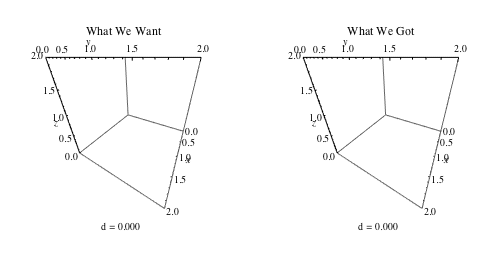If you watch the animation above where $n = (1,1,1)$, there’s a time where what we want is what we got. It works for $0 \le d < 1$, but for $d \ge 1$ the shape of the plane cut ceases to be a triangle and becomes a hexagon.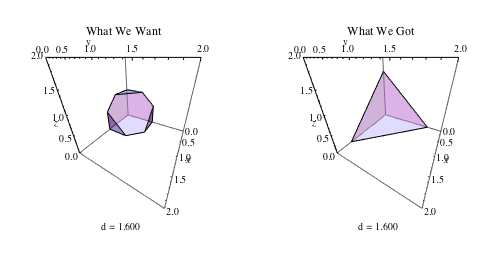So now what do we do? Should we try to redo our integrals and add a more cases? If you want a challenge or to test your understanding, read no further and take a moment to try and figure out what you’d do next by yourself.

### Help From Stackexchange

Luckily we don’t have to revisit our integrals. We can use this insight from Achille Hui’s stackexchange answer which was extremely helpful in setting up this problem. Hui solved a special case of this problem where n = (1,1,1).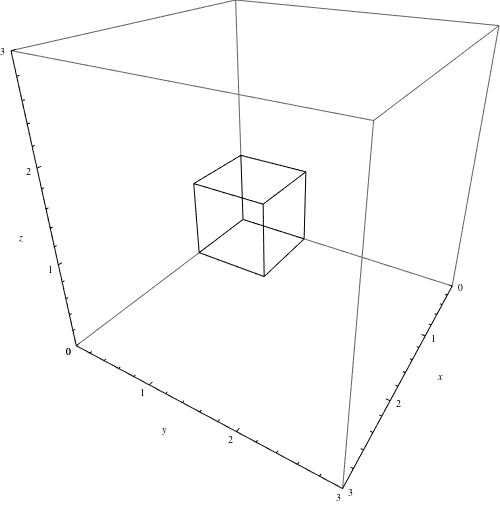Hui’s trick is to subtract off the parts that aren’t our cube. Since those parts are tetrahedrons, we’ve already derived the basic tools we need. In fact, you can think of it like we’re computing the volume of adjacent cubes translated by $\hat i, \hat j, \hat k$. This solution is recursive mathematically and programmatically! So we’ve got a solution for $1 \le d < 2$. What about $2 \le d \le 3$?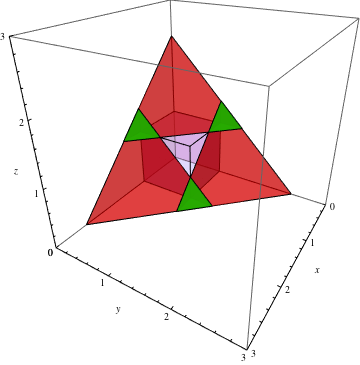When $d > 2$ the tetrahedrons that we’re subtracting start to overlap, but we’re now well equipped to deal with this. We’re double counting those subtractions so we add them back. You can think of this like we’re computing the volume of adjacent cubes translated by $\hat i + \hat j, \hat k + \hat i, \hat j + \hat k$.

## When to Correct?

With those couple tricks to correct our initial solution we’ve solved our problem conceptually. There are a few fiddly things left to do like determining when our corrections are necessary.

For instance, when do we have to subtract for the $x$ axis? When $x > 1$ and $y = z = 0$, we’ve got a correction to make. We go back to the plane equation plug in $x,y = 0$ and solve for $x = d/a$ then use the Heaviside step or unit step function $H(x)$ to only subtract the correction only when necessary. We do likewise for our over counting correction.

## Solution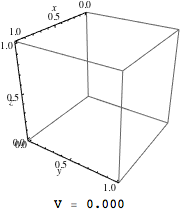Our final solution looks like this mathematically.

\begin{align} V_1(n, p_0) &= \frac{d^3}{6 a b c} \\ V &= V_1(n, p_0) \\ &- \sum_{i = 1}^3 H(d/n_i + 1) V_1(n, p_0 - e_i) \\ &+ \sum_{i = 1}^3 H((d - n_i)/n_{i + 1} - 1) V_1(n, p_0 - (e_i + e_{i + 1}) \\ H(x) &= \begin{cases} 0 & x < 0 \\ 1 & x \geq 0 \end{cases} \end{align}

This resolves our problem for determining the volume of a unit cube under a plane. A few transformations are needed to make it work for a cuboid of any dimensions. A few details are left out that have to do with covering all the cases. The code in the appendix does handle them.

## Applying this to Buoyancy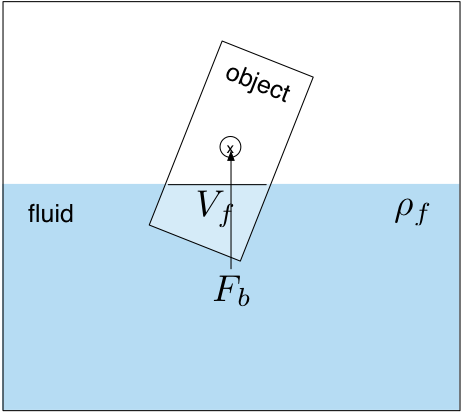We can apply this solution to simulate buoyancy in an object, and it will cause the object to rise, fall, or float in the fluid as you’d expect. However, we’ve made no mention about where this force ought to be applied. If it’s applied to the center of mass, your object will float but it won’t look right. A long plank of wood might float with its long end sticking straight out, which doesn’t happen in real life. It ought to fall over. It ought to rotate, but that won’t happen because the force is applied to the center of mass.

So what happens in real life? The buoyancy force is applied to the centroid of volume not the center of mass.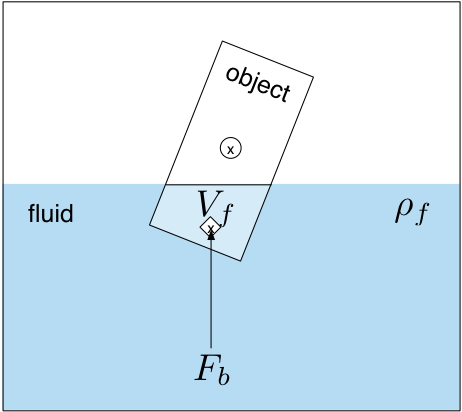This can induce a torque and will cause the object to rotate into a more natural orientation. Because the centroid of volume changes, the torque may oscillate; this is why many floating objects often seem to wobble. The derivation for centroid of volume is very similar to what we did above with a slight twist. We’ve done all the hard work already, but I’ll save that derivation for part 2. See the code or references ‘till then.

Thanks for reading. Let me know if I’ve made any glaring errors. If you’re interested in using this in Unity, ping me on twitter @shanecelis.

## Here’s a Cute Cat

Congratulations! You made it through this whole post. I know it was math heavy. You deserve a cute kitty!# Appendix: Code

I solved this problem in Mathematica. Having math and visualization tools close at hand made the problem easier to work with.

## Volume No Correction

This calculates the volume of a tetrahedron.

volumeNoCorrection[ng_, p0g_] := Module[{a, b, c, d, R, n, p0},
n = R . ng;
p0 = R . p0g;
d = n . p0;
a = n[]; b = n[]; c = n[];
If[zeroCount[n] == 0,
d^3/(6 a b c) ,
If[zeroCount[n] == 1,
d^2/(2 a c),
If[zeroCount[n] == 2,
d/c]]]]


## Volume

This calculates the volume of the unit cube correcting for the over and under counting.

volume[ng_, p0g_] := Module[{d, R, n, p0},
n = R . ng;
p0 = R . p0g;
d = n . p0;
If [d < 0,
0,
If[d > n . {1, 1, 1},
1,
volumeNoCorrection[n, p0]
- Sum[
If[n[[i]] == 0,
0,
UnitStep[d/n[[i]] - 1] volumeNoCorrection[n,
p0 - e[i] ]], {i, 1, 3}]
+ Sum[
If[n[[Mod[i + 1, 3, 1]]] == 0,
0,
UnitStep[(d - n[[i]])/n[[Mod[i + 1, 3, 1]]] -
1] volumeNoCorrection[n,
p0 - (e[i] + e[Mod[i + 1, 3, 1]] )]], {i, 1, 3}]
]
]
]


## Ancillary Code

Our integration expects a particular integration order: $z, x, y$. If the components of the normal are zero, we have to use a slightly different solution. This returns a transform. We want a transform because we may want to transform things back to the original space.

(* Return a transform matrix that will order the vertices by $n$'s zero components. Returns identity matrix if $n$ has no zeros. *)
reorderVertices[n_] := Module[{R, i},
If[zeroCount[n] == 0,
M0,
If[zeroCount[n] == 1,
i = Position[n, 0] // First // First;
R = {M3, M0, M1}[[i]] ;
R,
If[zeroCount[n] == 2,
i = Position[n, u_ /; u != 0] // First // First;
R = {M2, M1, M0}[[i]] ;
R]]]]

(* No swaps. *)
M0 = IdentityMatrix;
(* Swap z and y. *)
M1 = M0[[All, {1, 3, 2}]];
(* Swap z and x. *)
M2 = M0[[All, {3, 2, 1}]];
(* Swap x and y. *)
M3 = M0[[All, {2, 1, 3}]];

(* Return the number of zeros in a vector. *)
zeroCount[l_] := Count[l, u_ /; u === 0]

(* Returns a matrix that will transform the n vector to the first quadrant. *)